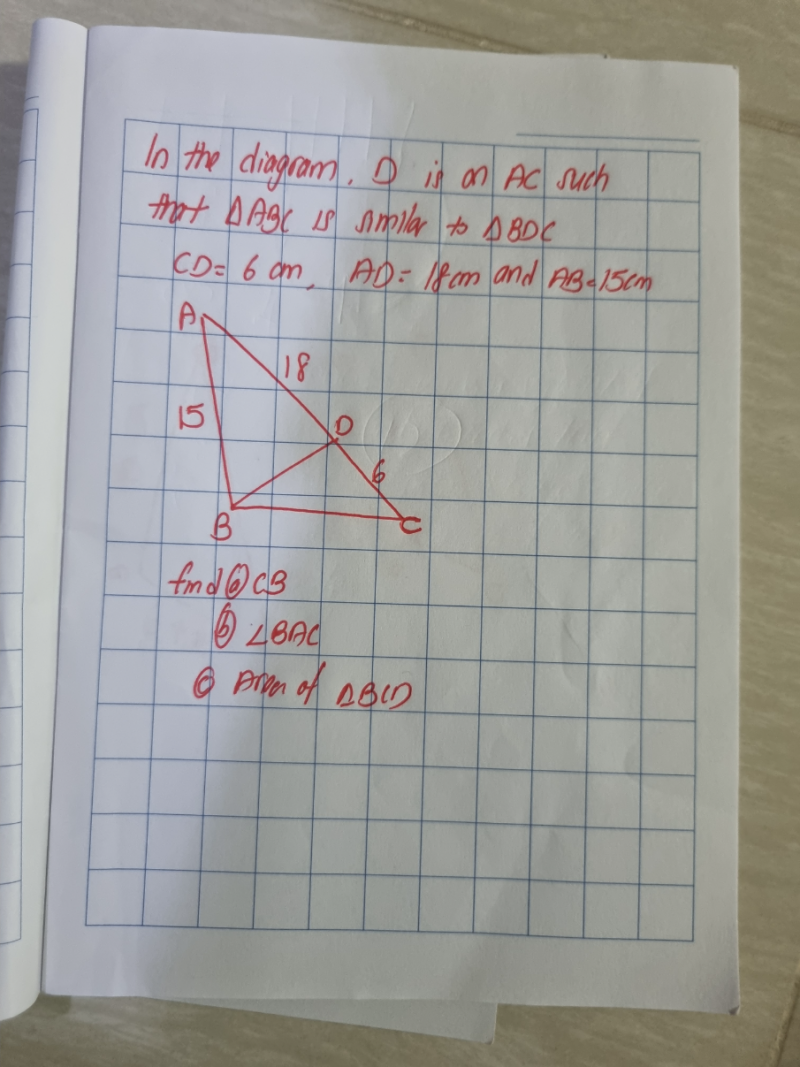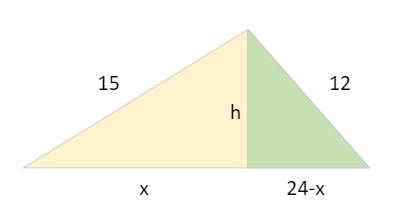# QuestionVery confusing of the triangle.pls help thanks.

(a)  Given △ABC is similar △BDC

Then    AB/BD = BC/DC = AC/BC

DC = CD = 6,   AD = 18   => AC =18+6 = 24

BC/6 = 24/BC

(BC)2 =24 x 6 = 144   => BC = CB = 12cm

(b)  Let Angle BAC = ∠Aa = BC = 12 , b = AC =24, c = AB = 15

Using Cosine Rulea = √[c2 + b2 – 2ab cos A] =>  a2 = [c2 + b2 – 2ab cos A]

– 2bc cos A = a –  c2 – b2

-(2)(24)(15) cos A = 144 – 225 – 576 = -657

cos A = 0.9125

∠A = Angle BAC = 24.150 (to 4 s.f.)

(c) Using the Sine Rule , Area of  △ABC = (1/2) bc sin A = (1/2)(24)(15) sin 24.15 = 73.64

Area of △BDC / Area of △ABC =  (BC/AC)

Area of △BDC = (12/24)2 x 73.64 = 18.4 cm (to 3 s.f.)

Thank you very much.

0 Replies 1 Like

sushi88 already gave an excellent answer. It is appropriate for Sec 3, where students should be aware of Cosine Rule and use it accordingly.

Below, for fun, I want to pretend I am only Sec 1 or 2 and only know definition of cosine and Pythagoras Theorem.

`Solution using Pythagoras Theorem and basic cosine````Part (b):
After part (a), we know triangle ABC has sides 15, 12 and 24.
Let h be the perpendicular. Then h2 is
152 - x2 = 122 - (24-x)2
152 - 122 = x2 - (24-x)2
27 * 3 = 24 * (2x - 24)   (difference of 2 squares on both sides!)
x = (81/24 + 24)/2 = 13.6875
So angle BAC = cos-1 (x/15) = 24.15 degrees (2 decimal places).

Part (c):
We don't even need angle BAC or knowledge of cosine. Just 1/2 base * height.
Area of ABC = 12 * h = 12 * sqrt(152 - x2)
= 73.6338 (4 decimal places)
Area of BCD = (6/12)**2 * Area ABC = 18.41 (2 decimal place)

Part (c) alternative:
In fact, if there was no Part (b), we can solve (c) using Heron's Formula.
The sides are a = 12, b = 15, c = 24. So s = (a+b+c)/2 = 25.5.
Area ABC = sqrt(s(s-a)(s-b)(s-c)) = 73.6338 (4 dp), etc.
Heron's Formula is probably not in the syllabus but it is useful to know. :)```
0 Replies 1 Like